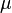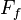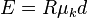# Friction

Friction is the force that opposes the relative motion or tendency of such motion of two surfaces in contact. It is not, however, a fundamental force, as it originates from the electromagnetic forces and exchange force between atoms. In situations where the surfaces in contact are moving relative to each other, the friction between the two objects converts kinetic energy into sensitive energy, or heat (atomic vibrations). Friction between solid objects and fluids (gases or liquids) is called fluid friction.

Friction is an extremely important force. For example, it allows us to walk on the ground without slipping, it helps propel automobiles and other ground transport, and it is involved in holding nails, screws, and nuts. On the other hand, friction also causes wear and tear on the materials in contact.

## Equations

The classical approximation of the force of friction, known as Coulomb friction (named after Charles-Augustin de Coulomb), is expressed as:$F_f = \mu R \,$,

where-$\mu$ is the coefficient of friction,$R$ is the reaction force normal to the contact surface,$F_f$ is the maximum possible force exerted by friction.

This force is exerted in the direction opposite the object's motion.

This law mathematically follows from the fact that contacting surfaces have atomically close contacts only over extremely small fraction of their overall surface area, and this contact area is proportional to load (until saturation which takes place when all area is in atomic contact thus no further increase of friction force takes place).

This simple (although incomplete) representation of friction is adequate for the analysis of many physical systems.

## Coefficient of friction

The coefficient of friction (also known as the frictional coefficient) is a dimensionless scalar value which describes the ratio of the force of friction between two bodies and the force pressing them together. The coefficient of friction depends on the materials used—for example, ice on metal has a low coefficient of friction (they slide past each other easily), while rubber on pavement has a high coefficient of friction (they do not slide past each other easily). Coefficients of friction need not be less than 1—under good conditions, a tire on concrete may have a coefficient of friction of 1.7. Magnetically attractive surfaces can have very large friction coefficients, and, theoretically, glued or welded together surfaces have infinite friction coefficients.

Sliding (kinetic) friction and static friction are distinct concepts. For sliding friction, the force of friction does not vary with the area of contact between the two objects. This means that sliding friction does not depend on the size of the contact area.

When the surfaces are adhesive, Coulomb friction becomes a very poor approximation (for example, transparent tape resists sliding even when there is no normal force, or a negative normal force). In this case, the frictional force may depend on the area of contact. Some drag racing tires are adhesive in this way.

The force of friction is always exerted in a direction that opposes movement (for kinetic friction) or potential movement (for static friction) between the two surfaces. For example, a curling stone sliding along the ice experiences a static force slowing it down. For an example of potential movement, the drive wheels of an accelerating car experience a frictional force pointing forward; if they did not, the wheels would spin, and the rubber would slide backwards along the pavement. Note that it is not the direction of movement of the vehicle they oppose but the direction of (potential) sliding between tire and road.

The coefficient of friction is an empirical measurement—it has to be measured experimentally, and cannot be found through calculations. Rougher surfaces tend to have higher values. Most dry materials in combination give friction coefficient values from 0.3 to 0.6. It is difficult to maintain values outside this range. A value of 0.0 would mean there is no friction at all. Rubber in contact with other surfaces can yield friction coefficients from 1.0 to 2.0.

The coefficient of friction, when multiplied by the reaction force on the object by the contact surface, will give the maximum frictional force opposing sliding on the object. However, if the force pulling on the object is less than the maximum force of friction then the force of friction will be equal to the force pulling on the object. You have to pull with a force greater than the maximum value of friction to move the object.

## Types of friction

Frictional force in mechanics is the force which opposes the relative motion of an object. It is directed opposite to the motion of an object.

There are three types of frictional forces.

1. Static friction is the friction acting on a body when the body is not in motion, but when a force is acting on it. Static friction is the same as the force being applied (because the body isn't moving). Static friction acts because the body tends to move when a force is applied on it.
2. Limiting friction is the friction on a body just before it starts moving. Generally, limiting friction is highest.
3. Kinetic friction is the friction which acts on the body when the body is moving. Kinetic friction is usually smaller than limiting friction.

The kinetic frictional force of a solid-solid interface is given by:$\ F=\mu R$

where R is the normal reaction force acting between the interface and the object and$\ \mu$ is the coefficient of kinetic friction. The value of the coefficient depends upon the nature of the surfaces.

The limiting friction is given by$\ F=\mu_{max} R$

where R is the normal reaction force acting between the interface and the object and$\ \mu_{max}$ is the coefficient of limiting friction.

For a fluid, the frictional force is directly proportional to the velocity of the object.

### Static friction

Static friction occurs when the two objects are not moving relative to each other (like a book on a desk). The coefficient of static friction is typically denoted as μs. The initial force to get an object moving is often dominated by static friction. The static friction is in most cases higher than the kinetic friction.

Examples of static friction:

Rolling friction occurs when one object "rolls" on another (like a car's wheels on the ground). This is classified under static friction because the patch of the tire in contact with the ground, at any point while the tire spins, is stationary relative to the ground. The coefficient of rolling friction is typically denoted as μr.

Limiting friction is the maximum value of static friction, or the force of friction that acts when a body is just on the verge of motion on a surface.

### Kinetic friction

Kinetic (or dynamic) friction occurs when two objects are moving relative to each other and rub together (like a sled on the ground). The coefficient of kinetic friction is typically denoted as μk, and is usually less than the coefficient of static friction. From the mathematical point of view, however, the difference between static and kinetic friction is of minor importance: take a coefficient of friction that depends on the sliding velocity and is such that its value at 0 (the static friction μs ) is the limit of the kinetic friction μk for the velocity tending to zero. Then a solution of the contact problem with such Coulomb friction solves also the problem with the original μk and any static friction greater than that limit.

Since friction is always exerted in a direction that opposes movement, kinetic friction always does negative work.

Examples of kinetic friction:

• Sliding friction is when two objects are rubbing against each other. Putting a book flat on a desk and moving it around is an example of sliding friction
• Fluid friction is the friction between a solid object as it moves through a liquid or a gas. The drag of air on an airplane or of water on a swimmer are two examples of fluid friction.

## Reducing friction

### Devices

Devices such as ball bearings or rollers can change sliding friction into much smaller rolling friction by reducing the points of contact on the object.

### Techniques

One technique used by railroad engineers is to back up the train to create slack in the linkages between cars. This allows the locomotive to pull forward and only take on the static friction of one car at a time, instead of all cars at once, thus spreading the static frictional force out over time.

Generally, when moving an object over a distance: To minimize work against static friction, the movement is performed in a single interval, if possible. To minimize work against kinetic friction, the movement is performed at the lowest velocity that's practical. This also minimizes frictional stress.

### Lubricants

A common way to reduce friction is by using a lubricant, such as oil or water, that is placed between the two surfaces, often dramatically lessening the coefficient of friction. The science of friction and lubrication is called tribology. Lubricant technology is when lubricants are mixed with the application of science, especially to industrial or commercial objectives.

Superlubricity, a recently-discovered effect, has been observed in graphite. It is the substantial decrease of friction between two sliding objects, approaching zero levels (a very small amount of frictional energy would still be dissipated).

Lubricants to overcome friction need not always be thin, turbulent fluids or powdery solids such as graphite and talc; acoustic lubrication actually uses sound as a lubricant.

## Energy of friction

According to the law of conservation of energy, no energy is destroyed due to friction, though it may be lost to the system of concern. Energy is transformed from other forms into heat. A sliding hockey puck comes to rest due to friction as its kinetic energy changes into heat. Since heat quickly dissipates, many early philosophers, including Aristotle, wrongly concluded that moving objects lose energy without a driving force.

When an object is pushed along a surface, the energy converted to heat is given by:$E = R\mu_k d \,$
where
R is the magnitude of the normal reaction force,
μk is the coefficient of kinetic friction,
d is the distance traveled by the object while in contact with the surface.

Physical deformation is associated with friction. While this can be beneficial, as in polishing, it is often a problem, as the materials are worn away, and may no longer hold the specified tolerances.

The work done by friction can translate into deformation and heat that in the long run may affect the surface's specification and the coefficient of friction itself. Friction can, in some cases, cause solid materials to melt.